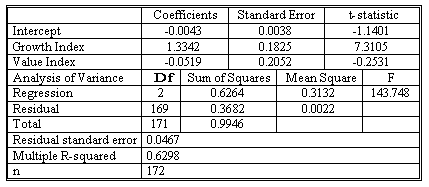### CFA Practice Question

There are 208 practice questions for this study session.

### CFA Practice Question

Suppose you want to know whether Fidelity Select Technology Fund (FSTF) behaves more like a large-cap growth fund or a large-cap value fund. You want to estimate the regression Y-hatt = b0 + b1X1t + b2X2t + et, where Yt is the monthly return to the FSTF, X1t is the monthly return to the S&P 500 Growth Index, and X2t is the monthly return to the S&P 500 Value index. The table below shows the results of a multiple linear regression using monthly data from December 1994 through March 2009.Calculate the standard error of estimate (SEE) for the regression in the table above.
Correct Answer: From the table we can calculate the standard error of estimate as (0.3682/169)1/2 = 0.0467. Thus the residual standard error is 4.67% a month.